Previous Page | Next Page

 Language Reference

## CHAR Function

produces a character representation of a numeric matrix

CHAR( matrix<,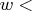,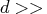)

The inputs to the CHAR function are as follows:

matrix
is a numeric matrix or literal.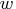is the field width.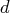is the number of decimal positions.
The CHAR function takes a numeric matrix as an argument and, optionally, a field widthand a number of decimal positions. The CHAR function produces a character matrix with dimensions that are the same as the dimensions of the argument matrix and with elements that are character representations of the corresponding numeric elements.

The CHAR function can take one, two, or three arguments. The first argument is the name of a numeric matrix and must always be supplied. The second argument is the field width of the result. If the second argument is not supplied, the system default field width is used. The third argument is the number of decimal positions in the result. If no third argument is supplied, the best representation is used. See also the description of the NUM function, which does the reverse conversion.

For example, the following statements produce the matrix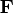, as shown:

```
a={1 2 3 4};
f=char(a,4,1);
```

```
F             1 row       4 cols    (character, size 4)

1.0  2.0  3.0  4.0
```Previous Page | Next Page | Top of Page